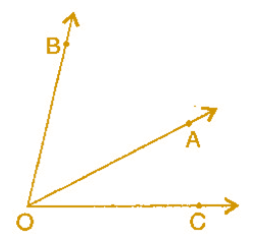# Ncert Class 6 Math Basic Geometrical Ideas Exercise 4.3

Basic Geometrical Ideas Class 6 Ex. 4.3

Ncert Class 6 Math Free Solution.

Exercise 4.3

Question 1 :- Name the angles in the given figure.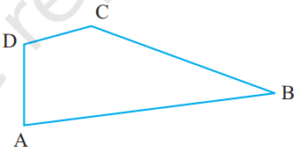Solution 1:-
The angles are:
(i) A or DAB
(ii) B or ∠ABC
(iii) C or ∠BCD
(iv) D or ∠CDA.

Question 2 :- In the given diagram, name the point(s):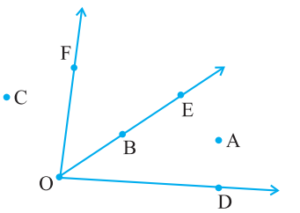(a) In the interior of ∠DOE
(b) In the exterior of ∠EOF
(c) On ∠EOF

Solution 2:-
(a) A is the point in the interior DOE.
(b) A, C, D is the point in the exterior EOF.
(c) E, B, O, F is the point on EOF.

Question 3 :- Draw rough diagrams of two angles such that they have
(а) one point in common.
(b) two points in common.
(c) three points in common.
(d) four points in common.
(e) One ray in common.

Solution 3:-
(a)  O is the common point of AOB and COB.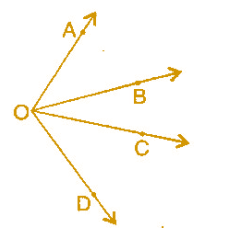(b) In figure, O and P are the common points in SOA and OPQ.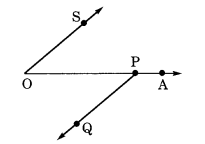(c) ∠ABF  and ∠EBF point B, D, F common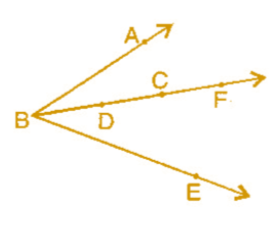(d) ∠BOA and ∠EOA  points O, D, C, A are common.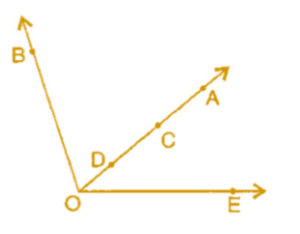(e) OA is the common ray of BOA and ∠COA.